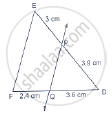Share

Books Shortlist
Your shortlist is empty

# Solution for In Below Fig., State If Pq || Ef. - CBSE Class 10 - Mathematics

ConceptBasic Proportionality Theorem Or Thales Theorem

#### Question

In below Fig., state if PQ || EF.#### Solution

We have,

DP = 3.9 cm, PE = 3cm, DQ = 3.6 cm and QF = 2.4 cm

Now,

"DP"/"PE"=3.9/3=1.3/1=13/10

And,

"DQ"/"QF"=3.6/2.4=36/24=3/2

rArr"DP"/"PE"!="DQ"/"QF"

So, PQ is not parallel to EF

Is there an error in this question or solution?

#### Video TutorialsVIEW ALL 

Solution for question: In Below Fig., State If Pq || Ef. concept: Basic Proportionality Theorem Or Thales Theorem. For the course CBSE
S